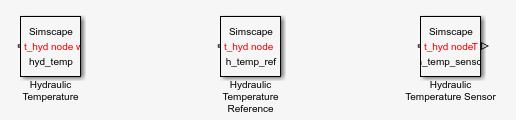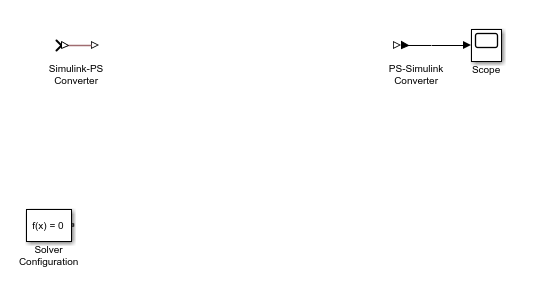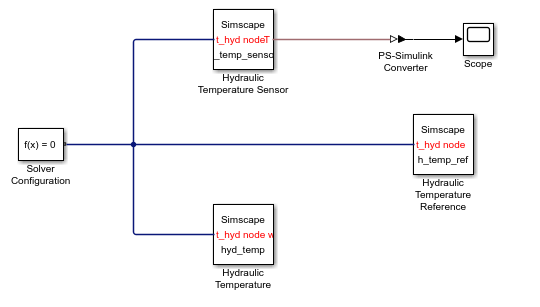## Working with Domain Parameters

### Declaring Domain Parameters

Similar to a component parameter, you declare each domain parameter as a value with unit. However, unlike component parameters, the main purpose of domain parameters is to propagate the same parameter value to all or some of the components connected to the domain.

### Propagation of Domain Parameters

The purpose of domain parameters is to propagate the same parameter value to all or some of the components connected to the domain. For example, this hydraulic domain contains one Across variable, `p`, one Through variable, `q`, and one parameter, `t`.

```domain t_hyd variables p = {1e6,'Pa'}; % pressure end variables(Balancing = true) q = {1e-3,'m^3/s'}; % flow rate end parameters t = {303,'K'}; % fluid temperature end end ```

All components with nodes connected to this domain will have access to the fluid temperature parameter `t`. The component examples in the following sections assume that this domain file, `t_hyd.ssc`, is located in a package named `+THyd`.

When dealing with domain parameters, there are three different types of components. There are some components that provide the domain parameter values used in the larger model, there are some that simply propagate the parameters, and there are some that do not propagate parameters.

For a complete example of building a custom block library based on this domain definition and using propagation of domain parameters in a simple circuit, see Custom Library with Propagation of Domain Parameters.

### Source Components

Source components provide a way to modify the domain parameter values. You declare a component parameter, and then use direct assignment to a domain parameter in the component node declaration. This assignment establishes the connection, which lets the parameter of the source component control the domain parameter value.

The following is an example of a source component, connected to the hydraulic domain `t_hyd`, defined in Propagation of Domain Parameters. This component provides the value of the temperature parameter to the rest of the model.

```component hyd_temp % Hydraulic Temperature % Provide hydraulic temperature to the rest of the model parameters t = {333,'K'}; % Fluid temperature end nodes a = THyd.t_hyd(t=t); % t_hyd node with direct parameter assignment end end ```

When you generate a Simscape™ block from this component file, the block dialog box will have a parameter labelled Fluid temperature. You can then use it to enter the temperature value for the hydraulic fluid used in the model. You cannot have more than one block controlling the same domain parameter connected to a circuit, unless different segments of the circuit are separated by a blocking component.

### Propagating Components

The default setting for the `Propagation` component attribute is `propagates`. Most components use this setting. If a component is configured to propagate its domain parameters, then all public nodes connected to this domain have the same set of domain parameters. These parameters are accessible in equations and other sections of the component file.

The following is an example of a propagating component `h_temp_sensor`, connected to the hydraulic domain `t_hyd`, defined in Propagation of Domain Parameters. It outputs the fluid temperature as a physical signal `T`. This example shows how you can access domain parameters in the equation section of a component.

```component h_temp_sensor % Hydraulic Temperature Sensor % Measure hydraulic temperature outputs T = {0,'K'}; % T:right end nodes a = THyd.t_hyd; % t_hyd node end equations T == a.t; % access parameter from node in equations end end ```

### Blocking Components

Blocking components are those components that do not propagate domain parameters. These components have their `Propagation` attribute set to `blocks`. If your model requires different values of a domain parameter in different segments of the same circuit, use blocking components to separate these segments and connect each segment to its own source component. For more information, see Attribute Lists.

### Custom Library with Propagation of Domain Parameters

The following example shows how you can test propagation of domain parameters by putting together a simple circuit. In this example, you will:

• Create the necessary domain and component files and organize them in a package. For more information, see Organizing Your Simscape Files.

• Build a custom block library based on these Simscape files. For more information, see Converting Your Simscape Files.

• Use these custom blocks to build a model and test propagation of domain parameters.

1. In a directory located on the MATLAB® path, create a directory called `+THyd`. This is your package directory, where you store all Simscape files created in the following steps.

2. Create the domain file `t_hyd.ssc`, as described in Propagation of Domain Parameters.

```domain t_hyd variables p = {1e6,'Pa'}; % pressure end variables(Balancing = true) q = {1e-3,'m^3/s'}; % flow rate end parameters t = {303,'K'}; % fluid temperature end end ```
3. Create the component file `hyd_temp.ssc`, as described in Source Components. This component provides the value of the temperature parameter to the rest of the model.

```component hyd_temp % Hydraulic Temperature % Provide hydraulic temperature to the rest of the model parameters t = {333,'K'}; % Fluid temperature end nodes a = THyd.t_hyd(t=t); % t_hyd node with direct parameter assignment end end ```
4. Create the component file `h_temp_sensor.ssc`, as described in Propagating Components. This component measures the value of the temperature parameter and outputs it as a physical signal.

```component h_temp_sensor % Hydraulic Temperature Sensor % Measure hydraulic temperature outputs T = {0,'K'}; % T:right end nodes a = THyd.t_hyd; % t_hyd node end equations T == a.t; % access parameter from node in equations end end ```
5. In order to create a working circuit, you will need a reference block corresponding to the domain type, as described in Grounding Rules. Create a reference component for your `t_hyd` domain, as follows (name the component `h_temp_ref.ssc`):

```component h_temp_ref % Hydraulic Temperature Reference % Provide reference for thermohydraulic circuits nodes a = THyd.t_hyd; % t_hyd node end connections connect(a,*); end end ```
6. You can optionally define other components referencing the `t_hyd` domain, but this basic set of components is enough to create a working circuit. Now you need to build a custom block library based on these Simscape files. To do this, at the MATLAB command prompt, type:

```ssc_build THyd; ```
7. This command generates a file called `THyd_lib` in the directory that contains your `+THyd` package. Before using this library, restart MATLAB to register the new domain. Then open the custom library by typing:

```THyd_lib ```8. Create a new Simscape model. To do this, type:

```ssc_new ```

This command creates a new model, prepopulated with the following blocks:9. Delete the Simulink-PS Converter block, because our model is not going to have any Simulink® input signals.

10. Drag the Hydraulic Temperature, Hydraulic Temperature Sensor, and Hydraulic Temperature Reference blocks from `THyd_lib` and connect them as follows:11. Simulate the model and notice that the scope displays the value of the domain temperature parameter, as it is defined in the `hyd_temp.ssc` file, 333 K.

12. Double-click the Hydraulic Temperature block. Change the value of the Fluid temperature parameter to `363` K.

13. Simulate the model again and notice that the scope now displays the new value of the domain temperature parameter.